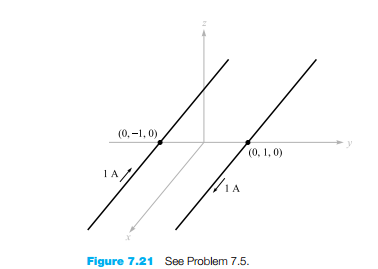### Create an Account

Already have account?

### Forgot Your Password ?

Home / Questions / Two circular current loops are centered on the z axis at z Each loop has radius a and carr...

# Two circular current loops are centered on the z axis at z Each loop has radius a and carries current I in the aφ direction a Find H on the z axis over the range

Two circular current loops are centered on the z axis at z = ±h. Each loop has radius a and carries current I in the aφ direction. (a) Find H on the z axis over the range −h(b) h = a/4; (c) h = a/2; (d) h = a. Which choice for h gives the most uniform field? These are called Helmholtz coils (of a single turn each in this case), and are used in providing uniform fields

Jun 14 2020 View more View Less

#### Answer (Solved)Subscribe To Get Solution